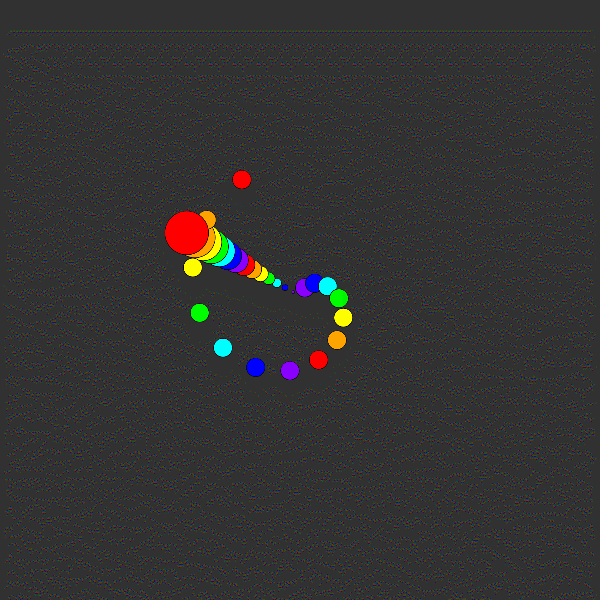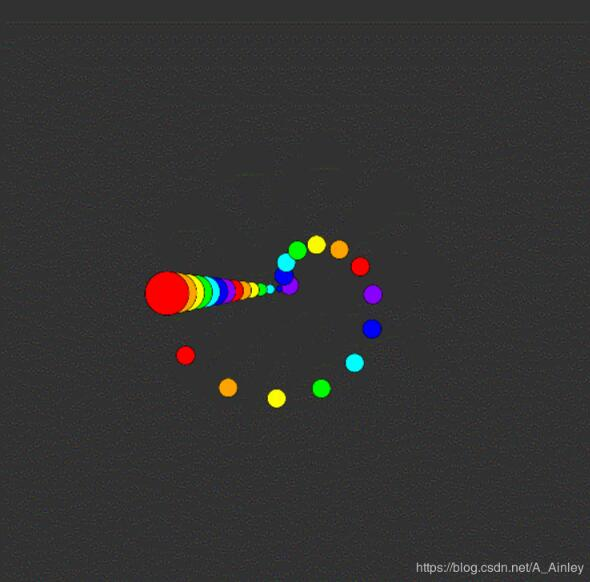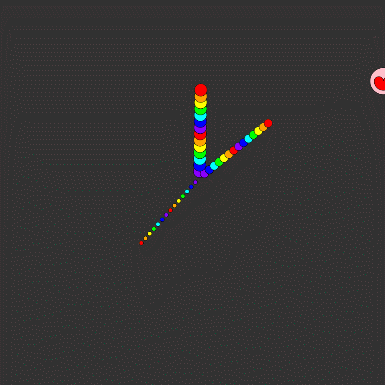# 使用p5.js實現動態GIF圖片臨摹重現

更新時間：2019年10月23日 14:21:11   作者：顧清宇我要評論1、觀察圖片可以看到，整個圖是由兩個部分組成的，其中一個是棍狀體，一個是螺旋狀體。

2、棍狀體從外到內越來越窄，整個圖形在做繞固定旋轉圓心的勻速圓周運動。

3、螺旋狀體也是在做繞固定旋轉圓心的勻速圓周運動。

4、螺旋狀體的旋轉角速度比棍狀體的旋轉角速度大。```//圓周運動棍狀體繪制函數
function drawBoll(r, g, b, interval)```

```//圓周運動螺旋體的繪制函數
function drawBoll2( r, g, b, interval)```

1、棍狀體：其實很簡單，我們可以看到靜止的棍狀體就是由14個小圓形組成的，由外到里半徑逐漸減小，然后顏色是赤橙黃綠青藍紫，赤橙黃綠青藍紫，每個圓形做的是繞固定圓心的勻速圓周運動。那么，我們的實現就是可以先做一個圓形的勻速圓周運動，其他的圓形，只需要修改一下旋轉半徑、圓形半徑以及顏色就可以了。至于圓周運動怎么實現，我會在后面講到，往后面看就可以了。

2、螺旋體：螺旋體其實也是一個圓形的重復變換得來的，可以看到的是，螺旋體的每個圓形都一樣大，不一樣的是什么呢？圓形的顏色和旋轉半徑，還有的就是他們初始就存在的弧度間隔。那么，我們要做的其實就是畫出一個勻速圓周運動的圓形，其它的就可以通過修改參數達到目的。

3、最后一步就是在draw()函數中去實現，我使用了一個for循環去實現：

```for (var i =1;i < 15; i++) // for循環實現變化的重復
{
//其中前三個參數是顏色的RGB值，最后一個參數i實現各個圓形之間的變化
drawBoll(139, 0, 255, i);
drawBoll2(139, 0, 255, i);
i++;
drawBoll(0, 0, 255, i);
drawBoll2(0, 0, 255, i);
i++;
drawBoll(0, 255, 255, i);
drawBoll2(0, 255, 255, i);
i++;
drawBoll(0, 255, 0, i);
drawBoll2(0, 255, 0, i);
i++;
drawBoll(255, 255, 0, i);
drawBoll2(255, 255, 0, i);
i++;
drawBoll(255, 165, 0, i);
drawBoll2(255, 165, 0, i);
i++;
drawBoll(255, 0, 0, i);
drawBoll2(255, 0, 0, i);
}```

```//準備畫板
function setup() {
createCanvas(1000, 1000);
}

//開始作畫
function draw() {
background(50); //背景顏色
for (var i =1;i < 15; i++) // for循環實現變化的重復
{
//其中前三個參數是顏色的RGB值，最后一個參數i實現各個圓形之間的變化
drawBoll(139, 0, 255, i);
drawBoll2(139, 0, 255, i);
i++;
drawBoll(0, 0, 255, i);
drawBoll2(0, 0, 255, i);
i++;
drawBoll(0, 255, 255, i);
drawBoll2(0, 255, 255, i);
i++;
drawBoll(0, 255, 0, i);
drawBoll2(0, 255, 0, i);
i++;
drawBoll(255, 255, 0, i);
drawBoll2(255, 255, 0, i);
i++;
drawBoll(255, 165, 0, i);
drawBoll2(255, 165, 0, i);
i++;
drawBoll(255, 0, 0, i);
drawBoll2(255, 0, 0, i);
}
}

//圓周運動棍狀體繪制函數
function drawBoll(r, g, b, interval){
fill(r, g, b);
var x = 500; //圓周運動圓心的x坐標
var y = 500; //圓周運動圓心的y坐標
var t = millis() / 3; // 控制旋轉速度的參數
var xChange //圓周運動x坐標變化值
var yChange; //圓周運動y坐標變化值
var radius = interval * 15;//圓周運動的半徑
xChange = radius * Math.cos(t * Math.PI/180);
yChange = radius * Math.sin(t * Math.PI/180);
x += xChange;
y += yChange;
ellipse(x, y, interval * 5, interval * 5);
}

//圓周運動螺旋體的繪制函數
function drawBoll2( r, g, b, interval){
fill(r, g, b);
var x = 500; //圓周運動圓心的x坐標
var y = 500; //圓周運動圓心的y坐標
var t = millis() / 1 + interval * 24; //24是兩個小球之間間隔的角度
var xChange //圓周運動x坐標變化值
var yChange; //圓周運動y坐標變化值
var radius = interval * 15;//圓周運動的半徑
xChange = radius * Math.cos(t * Math.PI/180);
yChange = radius * Math.sin(t * Math.PI/180);
x += xChange;
y += yChange;
ellipse(x, y, 30, 30);
}```

1、怎樣實現勻速圓周運動？

```var x = 500; //圓周運動圓心的x坐標
var y = 500; //圓周運動圓心的y坐標
var t = millis() / 3; // 控制旋轉速度的參數
var xChange //圓周運動x坐標變化值
var yChange; //圓周運動y坐標變化值
var radius = interval * 15;//圓周運動的半徑
xChange = radius * Math.cos(t * Math.PI/180);
yChange = radius * Math.sin(t * Math.PI/180);
x += xChange;
y += yChange;
ellipse(x, y, interval * 5, interval * 5);```

2、p5.js與之前接觸的編程語言的一些不同

`function drawBoll(r, g, b, interval)`

`function drawBoll(var r, var g, var b, var interval)````size = size + 3;
if (size > 50)
size = 20;
ellipse(mouseX, mouseY, 20+size, 20+size);

```

```size = size + 3;
if (size > 50)
size = 20;
fill(255,0,0);//紅色
arc(mouseX+size/4, mouseY, size/2, size/2, PI, 0);//半圓
arc(mouseX-size/4, mouseY, size/2, size/2, PI, 0);//半圓
arc(mouseX, mouseY, size, size, 0*PI, 1*PI);//半圓```

```//準備畫板
function setup() {
createCanvas(1000, 1000);
}

//開始作畫
function draw() {
background(50); //背景顏色
for (var i =1;i < 15; i++) // for循環實現變化的重復
{
//其中前三個參數是顏色的RGB值，最后一個參數i實現各個圓形之間的變化
drawBoll(139, 0, 255, i);
drawBoll2(139, 0, 255, i);
drawBoll3(139, 0, 255, i);
i++;
drawBoll(0, 0, 255, i);
drawBoll2(0, 0, 255, i);
drawBoll3(0, 0, 255, i);
i++;
drawBoll(0, 255, 255, i);
drawBoll2(0, 255, 255, i);
drawBoll3(0, 255, 255, i);
i++;
drawBoll(0, 255, 0, i);
drawBoll2(0, 255, 0, i);
drawBoll3(0, 255, 0, i);
i++;
drawBoll(255, 255, 0, i);
drawBoll2(255, 255, 0, i);
drawBoll3(255, 255, 0, i);
i++;
drawBoll(255, 165, 0, i);
drawBoll2(255, 165, 0, i);
drawBoll3(255, 165, 0, i);
i++;
drawBoll(255, 0, 0, i);
drawBoll2(255, 0, 0, i);
drawBoll3(255, 0, 0, i);
}
mouse(255, 192, 203);
}

//時針繪制函數
function drawBoll(r, g, b, interval){
fill(r, g, b);
var x = 500; //圓周運動圓心的x坐標
var y = 500; //圓周運動圓心的y坐標
var t = 6 * millis() / 3600000; // 控制旋轉速度的參數
var xChange //圓周運動x坐標變化值
var yChange; //圓周運動y坐標變化值
var radius = interval * 15;//圓周運動的半徑
xChange = radius * Math.cos(t * Math.PI/180 - Math.PI / 2);
yChange = radius * Math.sin(t * Math.PI/180 - Math.PI / 2);
x += xChange;
y += yChange;
ellipse(x, y, 30, 30);
}

//分針繪制函數
function drawBoll2( r, g, b, interval){
fill(r, g, b);
var x = 500; //圓周運動圓心的x坐標
var y = 500; //圓周運動圓心的y坐標
var t = 6 * millis() / 60000; // 控制旋轉速度的參數
var xChange //圓周運動x坐標變化值
var yChange; //圓周運動y坐標變化值
var radius = interval * 15;//圓周運動的半徑
xChange = radius * Math.cos(t * Math.PI/180 - Math.PI / 2);
yChange = radius * Math.sin(t * Math.PI/180 - Math.PI / 2);
x += xChange;
y += yChange;
ellipse(x, y, 20, 20);
}

//秒針繪制函數
function drawBoll3( r, g, b, interval){
fill(r, g, b);
var x = 500; //圓周運動圓心的x坐標
var y = 500; //圓周運動圓心的y坐標
var t = 6 * millis() / 1000; // 控制旋轉速度的參數
var xChange //圓周運動x坐標變化值
var yChange; //圓周運動y坐標變化值
var radius = interval * 15;//圓周運動的半徑
xChange = radius * Math.cos(t * Math.PI/180 - Math.PI / 2);
yChange = radius * Math.sin(t * Math.PI/180 - Math.PI / 2);
x += xChange;
y += yChange;
ellipse(x, y, 10, 10);
}

var size = 0;
// 實現鼠標交互的函數（律動的愛心）
function mouse(r, g, b){
fill(r, g, b);
size = size + 3;
if (size > 50)
size = 20;
ellipse(mouseX, mouseY, 20+size, 20+size);
fill(255,0,0);//紅色
arc(mouseX+size/4, mouseY, size/2, size/2, PI, 0);//半圓
arc(mouseX-size/4, mouseY, size/2, size/2, PI, 0);//半圓
arc(mouseX, mouseY, size, size, 0*PI, 1*PI);//半圓
}```

2019开奖结果